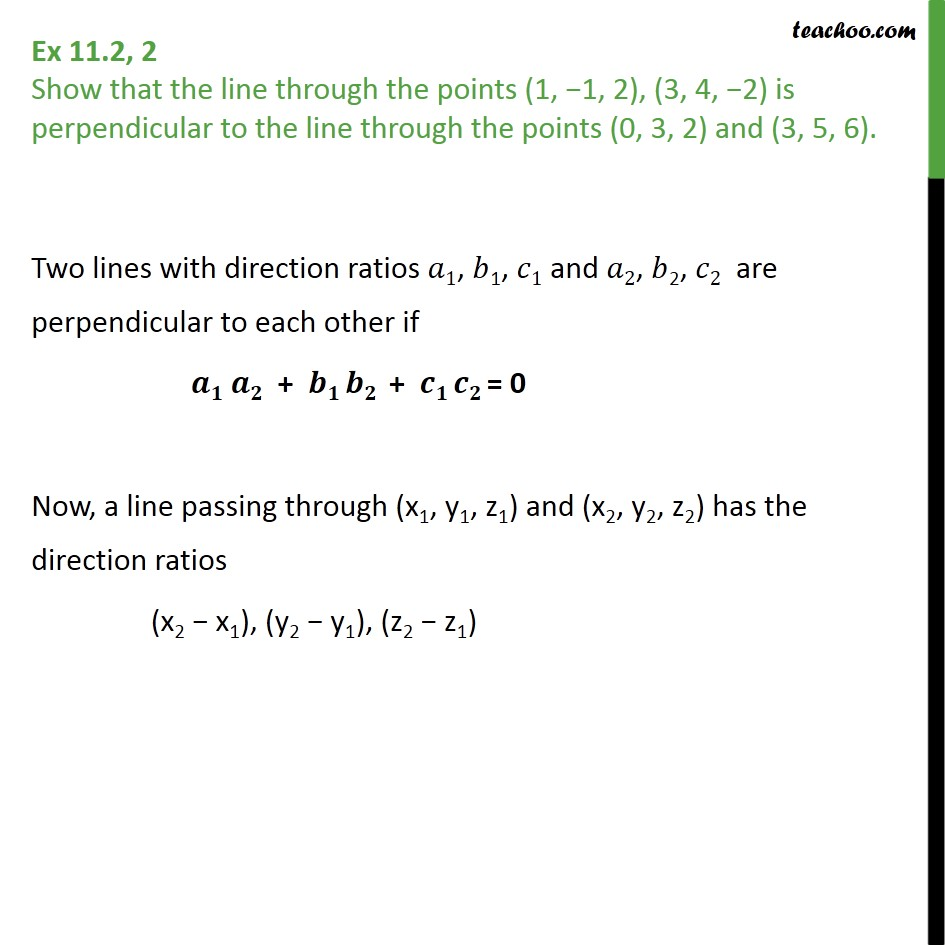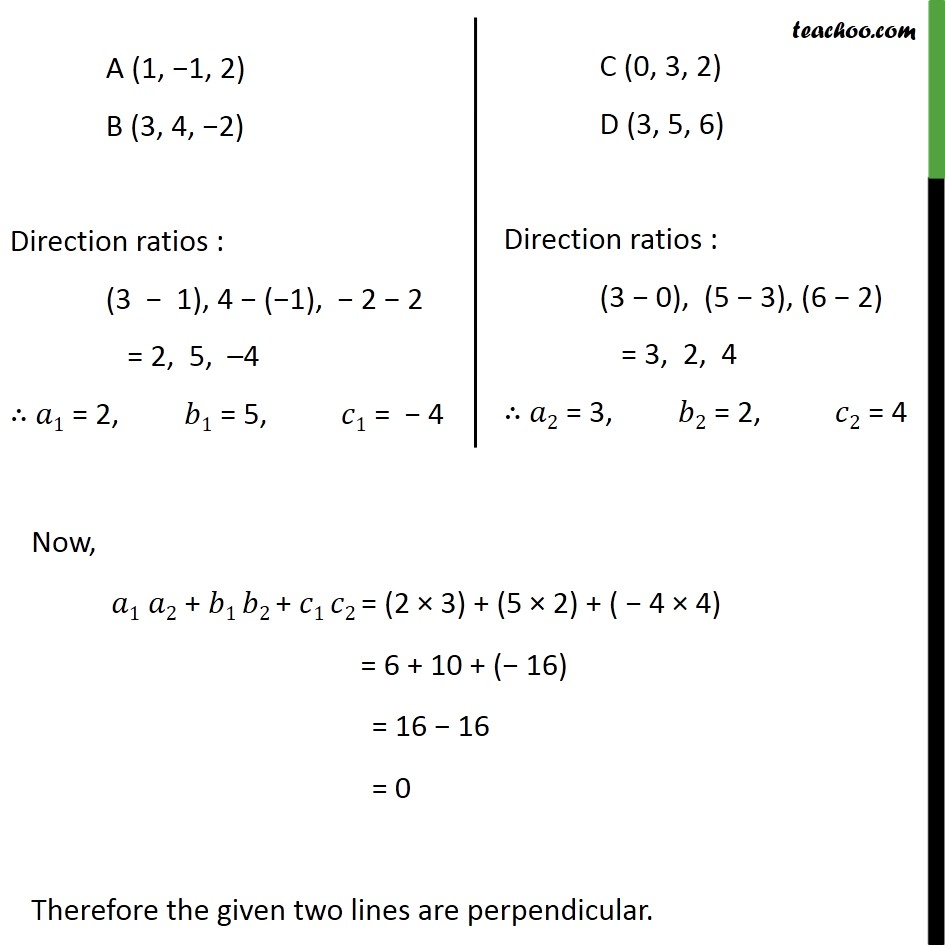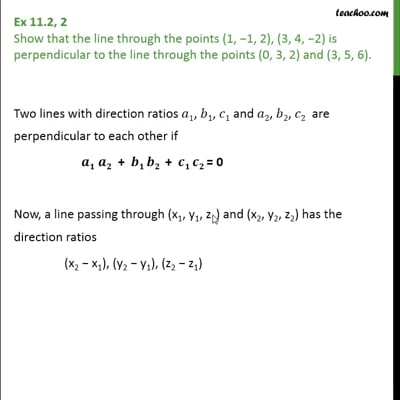Ex 11.2

Chapter 11 Class 12 Three Dimensional Geometry
Serial order wiseThis video is only available for Teachoo black users

Learn in your speed, with individual attention - Teachoo Maths 1-on-1 Class

### Transcript

Ex 11.2, 2 Show that the line through the points (1, 1, 2), (3, 4, 2) is perpendicular to the line through the points (0, 3, 2) and (3, 5, 6). Two lines with direction ratios 1, 1, 1 and 2, 2, 2 are perpendicular to each other if + + = 0 Now, a line passing through (x1, y1, z1) and (x2, y2, z2) has the direction ratios (x2 x1), (y2 y1), (z2 z1) Now, 1 2 + 1 2 + 1 2 = (2 3) + (5 2) + ( 4 4) = 6 + 10 + ( 16) = 16 16 = 0 Therefore the given two lines are perpendicular.